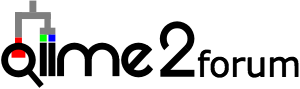# Mismatch between qiime2 taxa bar plot and phyloseq taxa bar plot

Hi all,

I know this isn’t 100% related to qiime2 since it also involves phyloseq so I am posting in the general discussion section.

I just discovered that the relative abundance plots I’ve made in phyloseq match none of my qiime2 taxa bar plots.

Here is an example.
Phyloseq:

qiime2:

I trust the qiime2 one more since it makes a lot more sense biologically. It appears that the microbiome diversity is getting “homogenized” after importing into phyloseq.

Here is my phyloseq code. I have tried making the plot pre filtering, post filtering, merging samples by metadata column in qiime2 and then importing, but nothing works.
Any ideas?

setwd(“C:/Users/samde/OneDrive - UCLA IT Services/Fish Project/ISLAND COMPILED PROJECT”)
destroyX(otu_table)
otu_table=as.matrix(otu_table)
taxonomy=as.matrix(taxonomy)
OTU=otu_table(otu_table, taxa_are_rows=TRUE)
TAX=tax_table(taxonomy)
#tree already imported as a phyloseq object
#merge into phyloseq object
isl=phyloseq(OTU,TAX,META,phy_tree)
isl

im <- merge_samples(islclean, “Tax”)
imr=rarefy_even_depth(im)
imr
imrt=transform_sample_counts(imr, function(x) x/sum(x))
ip=tax_glom(im,taxrank=“Phylum”)
ipt=transform_sample_counts(ip, function(x) x/sum(x))

imP=tax_glom(im,taxrank=“Phylum”)
imPf = filter_taxa(imP, function(x) sum(x > 0) > (0.000001*length(x)), TRUE)
imPf
imPfr=rarefy_even_depth(imPf)
imPfr
imPfrt=transform_sample_counts(imPfr, function(x) x/sum(x))

ip=plot_bar(imPfrt,fill=“Phylum”)
ip2=(ip+geom_bar(aes(fill=Phylum),stat=“identity”))
ip2

1 Like

We are missing some information on the QIIME 2 side, prior to making the taxonomy plot:

• What filtering was performed?
• Were the data rarefied ?
• Were unwanted taxa removed?
• That is, did you perform the same operations in QIIME 2 as you did in phyloseq? If not, you are truely comparing apples to oranges here.

Within R / phyloseq there are several operations that may be contributing to the observed differences:
You’ve:

• rarefied the data : `rarefy_even_depth(im)`
• ran `tax_glom(im,taxrank=“Phylum”)` w/o setting `NArm=False`
• This is likely the biggest issue. Any taxa with ‘NA’ in the column ‘Phylum’ (i.e. missing taxonomy for that rank) will be discarded unless you set this to `False`. Default is `True`. Check the help text for this.
• Finally you are removing low count reads: `filter_taxa(imP, function(x) sum(x > 0) > (0.000001*length(x)), TRUE)`

-I hope this helps!-Mike

3 Likes

Hi Mike,

Thanks for the feedback. So I reran the code on the unfiltered dataset this time to match the one from qiime2. I also confirmed that they both have 44,905 OTUs. I also ommitted the glom function to avoid any Unassigned issues. I get the exact same results. In qiime2 I simply ran the taxa bar plot function on the raw dataset so I think the only thing that occurs is transforming the OTUs to relative abundance correct? The only other thing I did in qiime was merge my samples by a metadata column prior to running the taxa bar plot (and I still get 44,905 OTUs after this).

So my phyloseq code is:

iht=phyloseq(OTU,TAX,META,phy_tree)
iht
pd <- psmelt(iht)

ipd<- ggplot(pd, mapping=aes(x = Sample, y = Abundance,fill=Phylum))
ipd2=( ipd +geom_bar(stat=“identity”)
+theme(legend.position=“none”)
)
ipd2

and my qiime code is

qiime taxa barplot
–i-table table.qza
–i-taxonomy taxonomy.qza
–o-visualization taxa-bar-plots.qzv

Here are the figures as well:
phyloseq:

qiime:

Note: You can see in the qiime2 figure that there should be some instances where Firmicutes takes up 50% of the sample abundance whereas in the phyloseq one you don’t even get close to that (see first fig). So even if the small reads or Unknowns were causing issues I don’t think there would be that big of a discrepancy right?

Thanks for this @Sam_Degregori, however I think the following command:

`ipd<- ggplot(pd, mapping=aes(x = Sample, y = Abundance,fill=Phylum))`

may suffer the same problems as the `tax_glom` command. I think one way to confirm this is to run a `forward fill` on the taxonomy data prior to plotting any of the data. There are a couple ways to do this, e.g. fill.forward, and fill. There may be others. This is the an optional strategy we use when constructing SILVA databases, see the RESCRIPt SILVA tutorial, and see this related but older post about forward filling taxonomy (i.e. propagation).

-Cheers!
-Mike

2 Likes